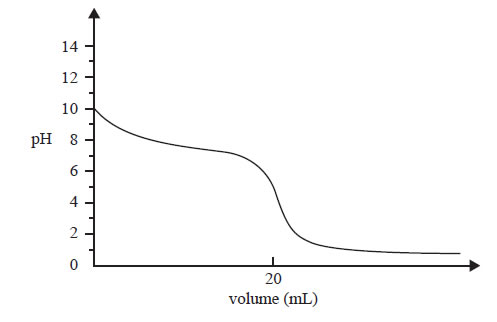General exercises (2010 VCE) The following titration curve was obtained by measuring the pH in a reaction flask during an acid-base titration.Question 1) The graph represents the change in pH in the reaction flask when A. a weak acid is added to a strong base. B. a weak base is added to a strong acid. C. a strong acid is added to a weak base. D. a strong base is added to a weak acid. Solution Question 2) Which one of the following is a suitable indicator for use in this titration? Click for the data sheet information. A. phenol red B. thymol blue C. phenolphthalein D. bromophenol blue SolutionQuestion 3) A sample of the insecticide dichlorodiphenyltrichloroethane (DDT), C14H9Cl5, was found to contain 0.120 g of carbon. What mass of chlorine was present in the sample? A. 0.127 g B. 0.355 g C. 0.994 g D. 1.01 g Solution Question 4 When 1.0 mole of Cu3FeS3 and 1.0 mole of O2 are mixed and allowed to react according to the equation 2Cu3FeS3(s) + 7O2(g) → 6Cu(s) + 2FeO(s) + 6SO2(g) A. no reagent is in excess. B. 5 mole of O2 is in excess. C. 5/7 mole of Cu3FeS3 is in excess. D. 2/7 mole of Cu3FeS3 is in excess. Solution Click to see the assessors report Question 5 One possible reaction that occurs when trinitrotoluene (TNT), C7H5N3O6, explodes is 2C7H5N3O6(s) → 2C(s) + 12CO(g) + 5H2(g) + 3N2(g) When one mole of TNT explodes the total volume of the gases produced from this reaction, measured at 27 °C and 1.00 × 102 kPa, is closest to A. 0.249 L B. 22.7 L C. 249 L D. 274 L Solution Click to see the assessors report